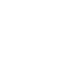# SAT & ACT Question of the Day: Jan. 22, 2014

If you are reading this in an email you received from me, do not click the link to sat.collegeboard.org below. Use the link to my website that is farther down on the email.

http://sat.collegeboard.org/practice/sat-question-of-the-day?questionId=20140122&oq=1 (This link takes you to today’s question. If you use my archive, you will see the question related to my SAT explanation for that date.)

The answer is A.  Wow, the test writer actually gave you a test-taking tip today.  That’s very rare.  Maybe they’ve been reading my blog and are learning how to take their own test!  They actually told you a good one but mine is still better.

Anytime you are faced with what looks like a lot of calculating, don’t do it.  There’s always a quick way to do the problem.  In this example, the denominator of one fraction cancels with the numerator of the following fraction.  That leaves you with 1/7.  However, that’s still the long way to do the question!  Keep reading.

I saw a very similar question in 1993.  You must think I have an incredible memory or am fabricating a story.  Actually, I remember it because I was at a College Board workshop and the presenter told the students to multiply all the numerators and then all the denominators and then reduce.  After they did all that work, I stood up and told them the Wizard’s strategy.  This question requires almost no math calculation.

What do you know about multiplying fractions?  That’s right–the product (answer) has to be smaller than the smallest fraction.  The smallest fraction is 1/2 in this problem; therefore, Answers C, D and E cannot be right because they are all larger than 1/2.  You can see that Answer B, 3/7, is way too big because 1/2 times 2/3 (1/2 of 2/3) is 1/3 (.33) which is smaller than 3/7 (approximately .43).  (I encourage you to memorize fraction/decimal equivalents.  I had to do that in elementary school and I use that information very frequently.  Thank you, Ms. Davis!)

What can you learn about the SAT (and ACT) from this question?  First, avoid doing lots of math calculations–you don’t need to.  Second, there will be many different ways to do the math and some will be much faster than others.  Finally, practice is critical because you’ll learn how to think about math and you will discover ways (by learning my strategies) that will make you much faster and a better math test taker.  Have fun.  These are game-playing strategies that will make your life a lot easier on test day.

Let’s see what the ACT folks have for us today.

Wonderful, today’s ACT question proves the point I was making above.

The answer is F.  Yes, you can use the Pythagorean Theorem.  8 squared is 64.  12 squared is 144.  Add them together and you’ll get 208.  Now what?  None of the answers look like 14.42 and that’s what you see on your calculator as the square root of 208.

Well, you could struggle with finding a perfect square as one of the factors of 208 which is 16, factor it out, and finally figure out A is the right answer.  If you really want to do it this way, start by squaring 4 and getting 16 to see if it is a factor of 208.  Why start with 4?  Look at the answers!  See all those 4’s?  That’s why!

Maybe an easier way for you to use your calculator is to multiply the answers to see which one comes out to 14.42.  For example, 4√10 is 12.64 and you know that is too small.  Because the answers are in descending (but usually ascending) order, you know A has to be right.

Or you could think about what you know and use it which will save your batteries and speed you up at the same time!  You know the hypotenuse has to be the longest side of the triangle; therefore, any answer less than 12 is wrong.  Get rid of H, J, and K.  You should know that the square root of 10 is only slightly longer than 3.  (The square root of 9 is 3.)  Therefore, Answer G is still too small because 4√10 is 12.64.  That leaves F:  4√13=14.42 which makes a lot more sense than 12.64, Answer G, which is only .64 inches longer than 12 (the long side).  Because the other side is 8, the hypotenuse will certainly be more than another .64 inches longer than the longer side (12).  To prove it to yourself just draw a diagram that is approximately to scale and you’ll see the evidence!

I know you are more comfortable with doing all the calculations for today’s SAT and ACT questions.  However, consider how much longer that took than just thinking for a few seconds.  These tests are timed and you need to go quickly so that you’ll have time to do the harder questions.  Practice and you’ll become more comfortable with my techniques and strategies.  You’ll figure out what works best for you.  It could turn out that you can do all the calculations and still finish on time.  In either case, your scores will improve.  However, you have to practice to figure out what works best for you.  Have fun–after all, you are playing a game!

The SAT & ACT Wizard